Aptitude Tests 4 Me

Non Verbal Logic

Detailed solution

150.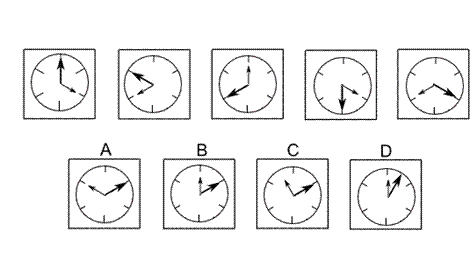Solution: B Explanation: In this question there is a dial with a big hand and a small hand. The big hand rotates about the centre of the dial by 60° anticlockwise each time. Following this rule, for the next diagram in the sequence, the big hand should point towards two o'clock. The correct answer, therefore, could be A, B or C. The small hand rotates about the centre of the dial by 120° clockwise each time. Following this rule, for the next diagram in the sequence, the small hand should point towards 12 o'clock. The correct answer, therefore, is B.

151.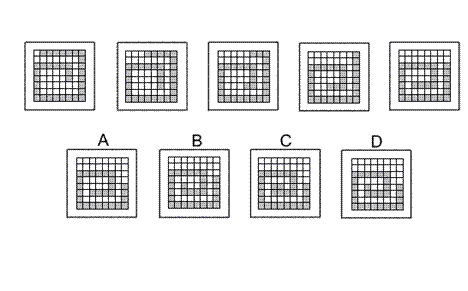Solution: D Explanation: In this question there is a 'snake' of grey squares that moves spiral-fashion in a clockwise sense towards the centre of the grid. As it moves, it gains one square at the 'head' of the 'snake' and loses two squares at the 'tail' of the 'snake' each time. The correct answer, therefore, is D.

152.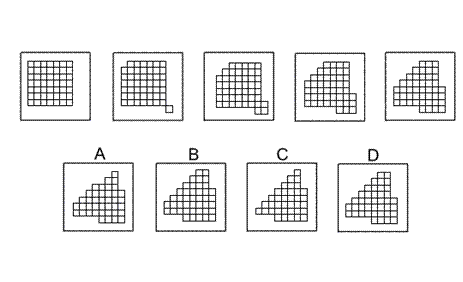Solution: B Explanation: In this question we start off with a 7 by 7 square grid. One square is removed from the top left corner of the grid and added to the bottom right corner of the grid to start an 'L-shape'. From then onwards, at each stage of the sequence, a diagonal of squares is removed from the top left hand corner of the grid and two squares are joined to the bottom right hand corner to increase the size of the 'Lshape'. Following this rule, for the next diagram of the sequence, a diagonal of five squares should be removed from the top left corner of the grid and two squares added to the bottom right corner to make a 5 by 5 'L-shape'. The correct answer, therefore, is B.

153.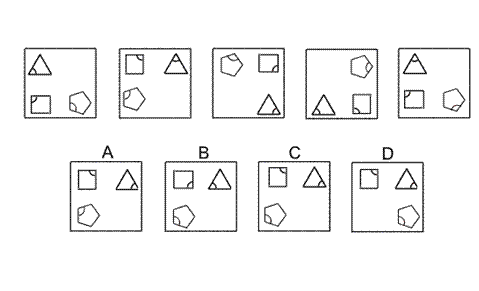Solution: C Explanation: In this question everything rotates clockwise. The equilateral triangle rotates about its own centre by 120° each time. The square rotates about its own centre by 90° each time. The regular pentagon rotates about its own centre by 108° each time. Simultaneously the three shapes rotate by 90° each time about the centre of the large square. Following these rules, the next diagram of the sequence must be C.

154.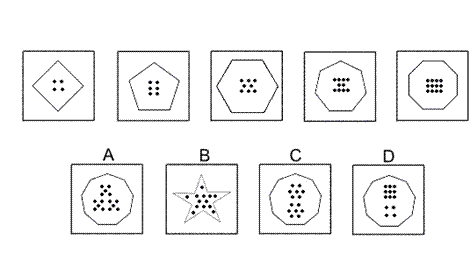Solution: C Explanation: In this question there are two rules to follow. The first rule is that the shapes are regular polygons, with the number of sides increasing by 1 each time. Following this rule, for the next diagram in the sequence, there should be a regular nonagon (nine-sided polygon). The correct answer, therefore, could be A, C or D. The other rule is that the number of dots inside the polygons increases by two each time. For the next diagram in the sequence, therefore, there should be 14 dots. The correct answer, therefore, is C.

 1 2 3 4 5 6 7 8 9 10 11 12 13 14 15 16 17 18 19 20 21 22 23 24 25 26 27 28 29 30 31 32 33 34 35 36 37 38 39 40 41 42 43 44 45 46 47 48 49 50 51 52 53 54 55 56 57 58 59 60 61 62 63 64 65 66 67 68 69 70 71 72 73 74 75 76 77 78 79 80 81 82 83 84 85 86 87 88Passage ReadingVerbal LogicNon Verbal LogicNumerical LogicData InterpretationReasoningAnalytical AbilityBasic NumeracyAbout UsContactPrivacy PolicyMajor TestsFAQ